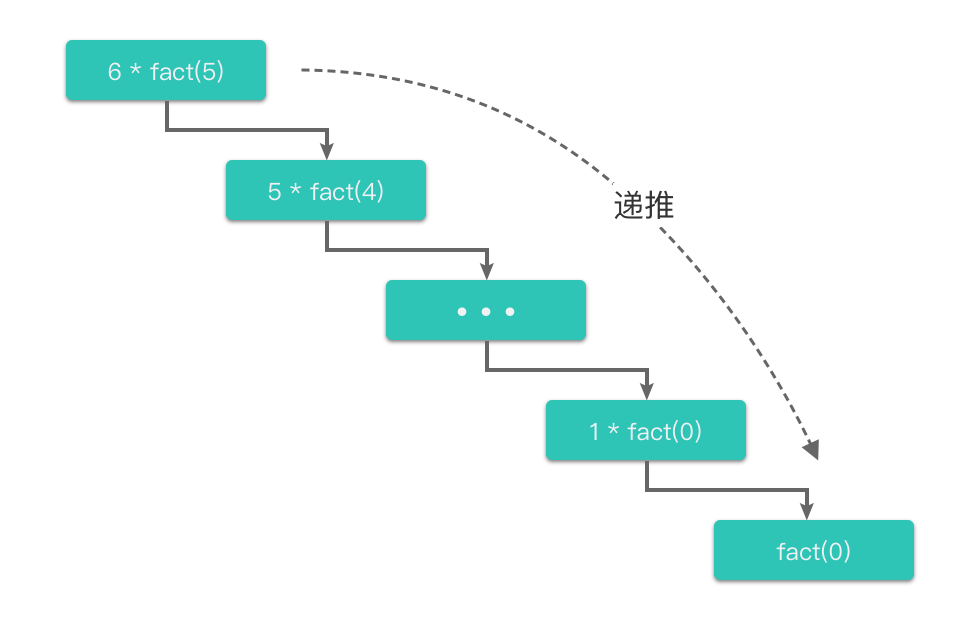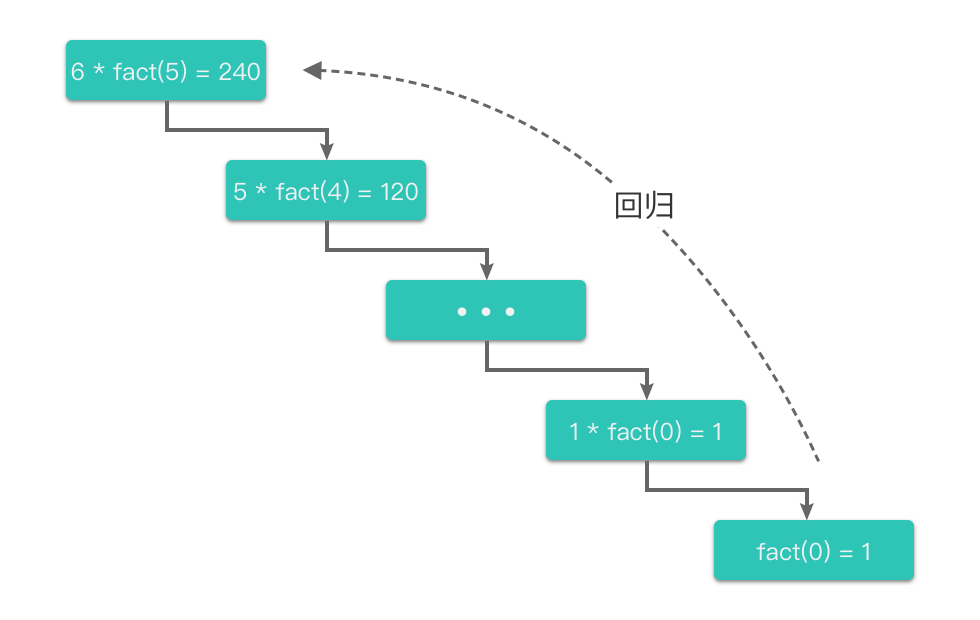01.递归算法知识

# 递归算法知识 #

## 1. 递归简介 #

$fact(n) = \begin{cases} 1 & \text{if n = 0} \cr n * fact(n - 1) & \text{if n > 0} \end{cases}$

 1 2 3 4  def fact(n): if n == 0: return 1 return n * fact(n - 1) 

n = 6 为例，上述代码中阶乘函数 fact(6) 的计算过程如下：

  1 2 3 4 5 6 7 8 9 10 11 12 13 14  fact(6) = 6 * fact(5) = 6 * (5 * fact(4)) = 6 * (5 * (4 * fact(3))) = 6 * (5 * (4 * (3 * fact(2)))) = 6 * (5 * (4 * (3 * (2 * fact(1))))) = 6 * (5 * (4 * (3 * (2 * (1 * fact(0)))))) = 6 * (5 * (4 * (3 * (2 * (1 * 1))))) = 6 * (5 * (4 * (3 * (2 * 1)))) = 6 * (5 * (4 * (3 * 2))) = 6 * (5 * (4 * 6)) = 6 * (5 * 24) = 6 * 120 = 720 

1. 函数从 fact(6) 开始，一层层地调用 fact(5)fact(4)、… 一直到最底层的 fact(0)
2. n == 0 时，fact(0) 不再继续调用自身，而是直接向上一层返回结果 1
3. fact(1) 通过下一层 fact(0) 的计算结果得出 fact(1) = 1 * 1 = 1，从而向上一层返回结果 1
4. fact(2) 通过下一层 fact(1) 的计算结果得出 fact(2) = 2 * 1 = 2 ，从而向上一层返回结果 2
5. fact(3) 通过下一层 fact(2) 的计算结果得出 fact(3) = 3 * 2 = 6 ，从而向上一层返回结果 6
6. fact(4) 通过下一层 fact(3) 的计算结果得出 fact(4) = 4 * 6 = 24，从而向上一层返回结果 24
7. fact(5) 通过下一层 fact(4) 的计算结果得出 fact(5) = 5 * 24 = 120，从而向上一层返回结果 120
8. fact(6) 通过下一层 fact(5) 的计算结果得出 fact(6) = 6 * 120 = 720，从而返回函数的最终结果 720

1. 先逐层向下调用自身，直到达到结束条件（即 n == 0）。
2. 然后再向上逐层返回结果，直到返回原问题的解（即返回 fact(6) == 720）。• 递推过程：指的是将原问题一层一层地分解为与原问题形式相同、规模更小的子问题，直到达到结束条件时停止，此时返回最底层子问题的解。
• 回归过程：指的是从最底层子问题的解开始，逆向逐一回归，最终达到递推开始时的原问题，返回原问题的解。

「递推过程」和「回归过程」是递归算法的精髓。从这个角度来理解递归，递归的基本思想就是： 把规模大的问题不断分解为子问题来解决。

## 2. 递归和数学归纳法 #

1. 证明当 n = bb 为基本情况，通常为 0 或者 1）时，命题成立。
2. 证明当 n > b 时，假设 n = k 时命题成立，那么可以推导出 n = k + 1 时命题成立。这一步不是直接证明的，而是先假设 n = k 时命题成立，利用这个条件，可以推论出 n = k + 1 时命题成立。

• 递归终止条件：数学归纳法第一步中的 n = b ，可以直接得出结果。
• 递推过程：数学归纳法第二步中的假设部分（假设 n = k 时命题成立），也就是假设我们当前已经知道了 n = k 时的计算结果。
• 回归过程：数学归纳法第二步中的推论部分（根据 n = k 推论出 n = k + 1），也就是根据下一层的结果，计算出上一层的结果。

## 3. 递归三步走 #

1. 写出递推公式：找到将原问题分解为子问题的规律，并且根据规律写出递推公式。
2. 明确终止条件：推敲出递归的终止条件，以及递归终止时的处理方法。
3. 将递推公式和终止条件翻译成代码
1. 定义递归函数（明确函数意义、传入参数、返回结果等）。
2. 书写递归主体（提取重复的逻辑，缩小问题规模）。
3. 明确递归终止条件（给出递归终止条件，以及递归终止时的处理方法）。

### 3.3 将递推公式和终止条件翻译成代码 #

1. 定义递归函数：明确函数意义、传入参数、返回结果等。
2. 书写递归主体：提取重复的逻辑，缩小问题规模。
3. 明确递归终止条件：给出递归终止条件，以及递归终止时的处理方法。

#### 3.3.4 递归伪代码 #

 1 2 3 4 5  def recursion(大规模问题): if 递归终止条件: 递归终止时的处理方法 return recursion(小规模问题) 

## 4. 递归的注意点 #

### 4.2 避免重复运算 ### 5. 递归的应用 #

### 5.1 斐波那契数 #

#### 5.1.2 题目大意 #

• 斐波那契数列的定义如下：
• f(0) = 0, f(1) = 1
• f(n) = f(n - 1) + f(n - 2)，其中 n > 1

 1 2 3  输入 n = 2 输出 1 解释 f(2) = f(1) + f(0) = 1 + 0 = 1 

#### 5.1.3 解题思路 #

1. 写出递推公式：f(n) = f(n - 1) + f(n - 2)
2. 明确终止条件：f(0) = 0, f(1) = 1
3. 翻译为递归代码：
1. 定义递归函数：fib(self, n) 表示输入参数为问题的规模 n，返回结果为第 n 个斐波那契数。
2. 书写递归主体：return self.fib(n - 1) + self.fib(n - 2)
3. 明确递归终止条件：
1. if n == 0: return 0
2. if n == 1: return 1

#### 5.1.4 代码 #

 1 2 3 4 5 6 7  class Solution: def fib(self, n: int) -> int: if n == 0: return 0 if n == 1: return 1 return self.fib(n - 1) + self.fib(n - 2) 

### 5.2 二叉树的最大深度 #

#### 5.2.2 题目大意 #

• 二叉树的深度：根节点到最远叶子节点的最长路径上的节点数。
• 叶子节点：没有子节点的节点。

 1 2 3 4 5 6 7 8 9  输入 [3,9,20,null,null,15,7] 对应二叉树 3 / \ 9 20 / \ 15 7 输出 3 解释 该二叉树的最大深度为 3 

#### 5.2.3 解题思路 #

1. 写出递推公式：当前二叉树的最大深度 = max(当前二叉树左子树的最大深度, 当前二叉树右子树的最大深度) + 1
• 即：先得到左右子树的高度，在计算当前节点的高度。
2. 明确终止条件：当前二叉树为空。
3. 翻译为递归代码：
1. 定义递归函数：maxDepth(self, root) 表示输入参数为二叉树的根节点 root，返回结果为该二叉树的最大深度。
2. 书写递归主体：return max(self.maxDepth(root.left) + self.maxDepth(root.right))
3. 明确递归终止条件：if not root: return 0

#### 5.2.4 代码 #

 1 2 3 4 5 6  class Solution: def maxDepth(self, root: Optional[TreeNode]) -> int: if not root: return 0 return max(self.maxDepth(root.left), self.maxDepth(root.right)) + 1